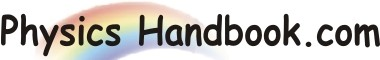HOME TOPICS DEFINITIONS TABLES LAWS INVENTIONS EXPERIMENTS QUIZ VIDEOS
 Physics Definitions Magnetic Field : The region around a current carrying conductor (or a magnet) where magnetic effects can be experienced is called magnetic field. Mutual Inductance : The property of two coils by virtue of which each oppose any change of current flowing in the other is called mutual inductance between two coils. Mass Number : The total number of nucleons (protons plus neutrons) called mass number (or atomic mass number) and is represented by the symbol A. This name is used since the mass of a nucleus is very closely A times the mass of one nucleon. A nucleus with 7 protons and 8 neutrons thus has Z = 7 and A = 15.Clearly, neutron number, N = A - Z. Mass Defect : The difference between the actual mass of the nucleus and the sum of masses of the constituent nucleons is called mass defect i.e. Mass Defect, Δm = M - A. Modulation Factor : The ratio of change of amplitude of carrier wave to the amplitude of normal carrier wave is called the modulation factor ma i.e. modulation factor ma = Amplitude change of carrier wave / Normal carrier wave (unmodulated) Milky Way : The galaxy to which sun belongs is called milky way i.e. this is the galaxy in which we live.